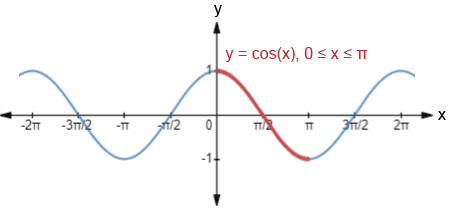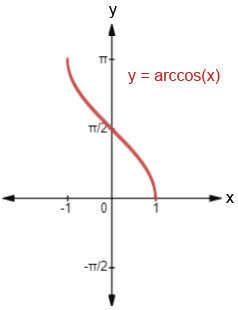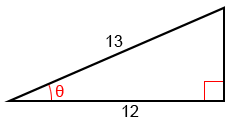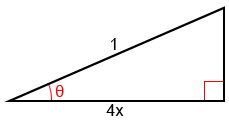# Arccos

Arccosine, written as arccos or cos-1 (not to be confused with), is the inverse cosine function. Both arccos and cos-1 are the same thing. Cosine only has an inverse on a restricted domain, 0 ≤ x ≤ π. In the figure below, the portion of the graph highlighted in red shows the portion of the graph of cos(x) that has an inverse.The domain must be restricted because in order for a function to have an inverse, the function must be one-to-one, meaning that no horizontal line can intersect the graph of the function more than once. Since cosine is a periodic function, without restricting the domain, a horizontal line would intersect the function periodically, infinitely many times.

One of the properties of inverse functions is that if a point (a, b) is on the graph of f, the point (b, a) is on the graph of its inverse. This effectively means that the graph of the inverse function is a reflection of the graph of the function across the line y = x.

The graph of y = arccos(x) is shown below.As can be seen from the figure, y = arccos(x) is a reflection of cos(x), given the restricted domain 0 ≤ x ≤ π, across the line y = x. The domain of arccos(x) is -1 ≤ x ≤ 1 and its range is 0 ≤ y ≤ π.

## Arccos calculator

The following is a calculator to find out either the arccos value of a number between -1 and 1 or cosine value of an angle.

## Arccos formula

The arccos formula is the inverse of the right triangle definition of the cosine function. Since, the arccos formula is therefore:## Using special angles to find arccos

While we can find the value of arccosine for any x value in the interval [-1, 1], there are certain angles that are used frequently in trigonometry (0°, 30°, 45°, 60°, 90°, and their multiples and radian equivalents) whose cosine and arccosine values may be worth memorizing. Below is a table showing these angles (θ) in degrees, and their respective cosine values, cos(θ).

 θ 0° 30° 45° 60° 90° 120° 135° 150° 180° cos(θ) 10-1

One method that may help with memorizing these values is to express all the values of cos(θ) as fractions involving a square root. Starting from 0° and progressing through 90°, cos(0°)=1=. The subsequent values, cos(30°), cos(45°), cos(60°), and cos(90°) follow a pattern such that using the value of cos(0°) as a reference, to find the values of cosine for the subsequent angles, we simply decrease the number under the radical sign in the numerator by 1, as shown below:

 θ 0° 30° 45° 60° 90° cos(θ)0

From 90° to 180°, we increase the number under the radical by 1 instead, but also must take into account the quadrant that the angle is in. Cosine is negative in quadrants II and III, so the values will be equal but negative. In quadrants I and IV, the values will be positive. This pattern repeats periodically for the respective angle measurements.

Once we've memorized the values, or if we have a reference of some sort, it becomes relatively simple to recognize and determine cosine or arccosine values for the special angles.

Example:

Find arccos() , arccos(), and arccos(2) in radians.,.,.

arccos(2) is undefined because 2 is not within the interval -1 ≤ arccos(θ) ≤ 1, the domain of arccos(x).

## Inverse properties

Generally, functions and their inverses exhibit the relationship

f(f-1(x)) = x   and   f-1(f(x)) = x

given that x is in the domain of the function. The same is true of cos(x) and arccos(x) within their respective restricted domains:

cos(arccos(x)) = x, for all x in [-1, 1]

and

arccos(cos(x)) = x, for all x in [0, π]

These properties allow us to evaluate the composition of trigonometric functions.

### Composition of arccosine and cosine

If x is within the domain, evaluating a composition of arccosine and cosine is relatively simple.

Examples:

1.2.If x is not within the domain, we need to determine the reference angle as well as the relevant quadrant. Given arccos(cos()), we cannot evaluate this as we did above because x is not within [0, π], so the solution cannot be. To evaluate this, we need to first determine cos() before using arccos:

3.In the above example, the reference angle is, and cos() is, but sincelies in quadrant III, its cosine is negative, and the only angle whose cosine is, that lies within the domain of arccos(x), is.

### Composition of other trigonometric functions

We can also make compositions using all the other trigonometric functions: sine, tangent, cosecant, secant, and cotangent.

Example:

Find sin(arccos()).

Sinceis not one of the ratios for the special angles, we can use a right triangle to find the value of this composition. Given arccos() = θ, we can find that cos(θ) =. The right triangle below shows θ and the ratio of its adjacent side to the triangle's hypotenuse.To find sine, we need to find the opposite side since sin(θ)=. Let a be the length of the opposite side. Using the Pythagorean theorem,

a2 + 122 = 132

a2 + 144 = 169

a2 = 25

a = 5

and

sin(arccos()) = sin(θ) =The same process can be used with a variable expression.

Example:

Find tan(arccos(4x)).

Given arccos(4x) = θ, we can find that cos(θ)=and construct the following right triangle:To find tangent, we need to find the opposite side, since tan(θ)=. Let b be the length of the opposite side. Using the Pythagorean theorem,

(4x)2 + b2 = 12

16x2 + b2 = 1

b2 = 1 - 16x2

b =and

tan(arccos(4x)) = tan(θ) =, where -< x <## Using arccosine to solve trigonometric equations

Arccosine can also be used to solve trigonometric equations involving the cosine function.

Example:

Solve the following trigonometric equations for x where 0 ≤ x < 2 π.

1. 2cos(x) =2cos(x) =cos(x) =x = arccos()

Cosine is negative in quadrants II and III, so there are two solutions: x =and x =. These are the only two angles within 0 ≤ x < 2π whose cosine value is equal to.

2. 6cos2(x) + 9cos(x) - 36 = 0

6cos2(x) + 9sin(x) - 6 = 0

(6cos(x) - 3)(cos(x) + 2) = 0

6cos(x) - 3 = 0 or cos(x) + 2 = 0

cos(x) =or cos(x) = -2

x = arccos() or x = arccos(-2)

Solving for x = arccos(),

x =orWe cannot solve for x = arccos(-2) because it is undefined, so x=orare the only solutions.